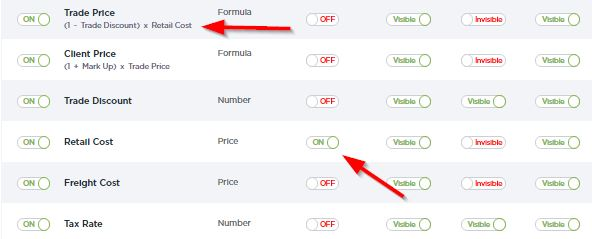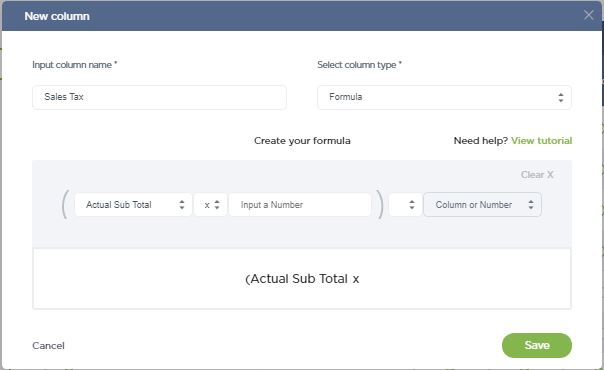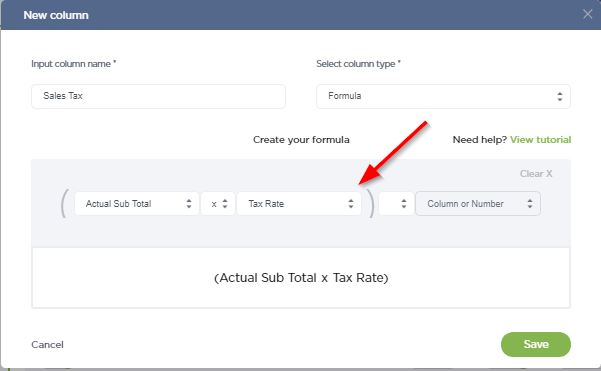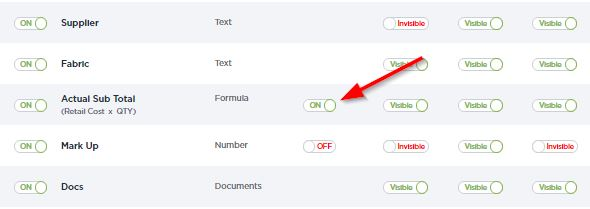# How to Configure Profit and Cost Calculation

Fohlio’s sample project template provides calculations of your product purchase cost, profit, etc. You can always customize the formula per each project’s needs. Here is how it works:

If you purchase on behalf of your client, we have some basic calculation setup for your reference in the Sample Project (With Purchasing), and you can change them to however it suits you. How each column is calculated is shown in the bracket.For example, you can always add new columns to include Sales Tax, shipping fees, etc. They can be setup as a formula like a sample here, Sales Tax = Actual Sub-Total * a number (your only and fixed tax rate number)Or you have different sales tax rate for different products, you can add a numeric column called Sales Tax Rate (in this column you can input different rate for each item), and then set the Sales Tax = Actual Sub-Total * Sales Tax RateYou can configure how to calculate the total cost number shown in the Organizer Folder and the presentation files.

The total cost is the sum of the all columns with the toggle on.If column A and column B are both turned on, then the total cost = sum of all numbers in column A  + sum of all numbers in column B.

For example, If you only have your quantity column turned on, we’ll display the total number of items (ex. 17 items).

If you have the cost of each item turned on, we’ll display the total cost of all of the items (ex. \$1,300.00) If you have both turned on, we’ll display your total cost plus how every many numbers (\$1317.00).

That last number is probably less than helpful.

SHARE+ this on: